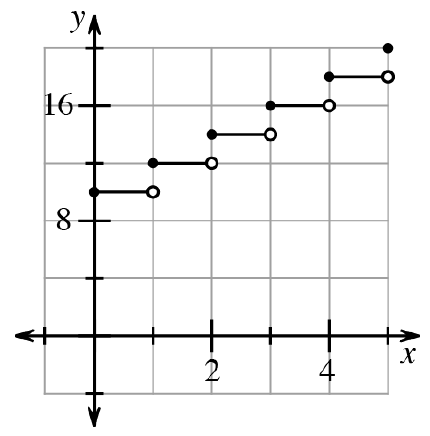### Home > CCA2 > Chapter Ch1 > Lesson 1.1.2 > Problem1-12

1-12.

Junior is saving money in his piggy bank. He starts with $10\ \text{cents}$ and adds two pennies each day. Create an $x→y$ table and a graph for the function for which $x$ represents the number of days since Junior started saving money and $y$ represents the total money he has saved. .

Make a table where $x$ represents the number of days and $y$ represents the total so far.

$10$ cents

How much did he have at the end of day 1? Day 2?...

$12,14,...$

What is the $y$-intercept? The slope?

$10,2$Completed the table in the eTool below to graph the function.
Click the link to the right for full version of the eTool: CCA2 1-12 HW eTool